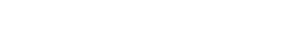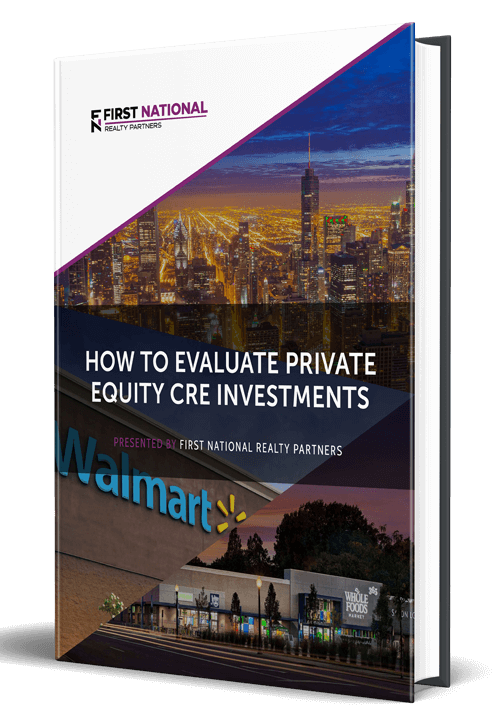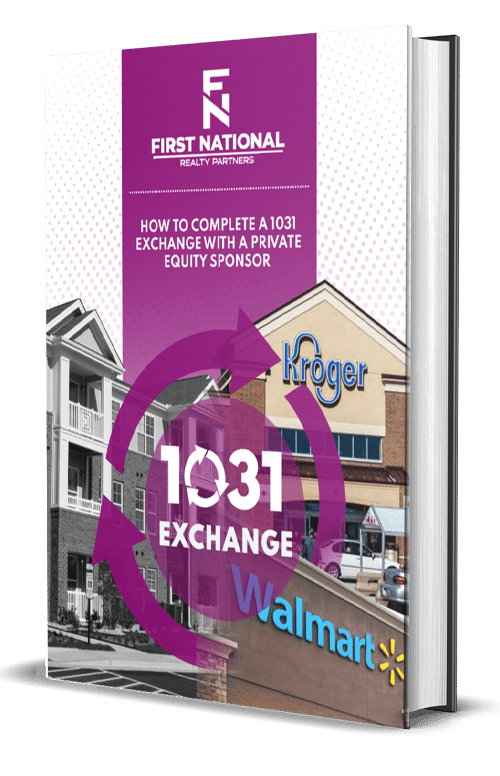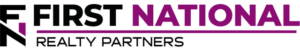# How to Calculate the Effective Annual Interest Rate in Commercial Real Estate

### Key Takeaways

• The effective annual rate is the rate of interest earned on an investment or paid on a loan given the effect of compounding over time.
• Calculating the effective annual rate of return is a useful means for comparing commercial real estate investment opportunities and loan products to each other.
• The effective annual rate calculation is a function of the stated interest rate and frequency of compounding.  The effective annual rate formula is:  Effective Annual Rate = 1 + ((Nominal Annual Interest Rate/# compounding periods) ^ # compounding periods)-1
• The greater the number of compounding periods, the higher the return.
• While the effective annual rate can be used for a wide variety of financial products, it can be useful for commercial real estate borrowers as a means of understanding the true cost of a loan and for commercial real estate investors as a means for understanding the true return.

Nearly every commercial real estate investment transaction involves both a loan and an investor who is expecting to earn a return on the capital they contribute to the purchase.  In both cases, the amount of interest paid on a loan or the return made on an investment is measured as an annual number.  While this is a valuable data point, it is perhaps too simplistic a measure because it does not account for the effect of compounding.  For this reason, many investors prefer to rely on a metric known as the Effective Annual Rate.

In this article, the Effective Annual Rate (EAR) is defined and an example is provided to inform readers about the utility of this metric.  By the end, readers will have a higher degree of comfort with this metric and will understand how to calculate it.

## What is the Effective Annual Rate?

The Effective Annual Rate is the rate of interest earned on a loan or commercial real estate investment as a result of compounding over time.  This metric is a helpful way to compare different loans or investments that compound over a different number of periods.

In most cases, the effective rate is higher than the “nominal” rate, which quotes an annual rate regardless of the number of compounding periods.  The more compounding periods, the higher the rate.

## How to Calculate the Effective Annual Rate

The formula used to calculate the effective annual rate is:

Effective Annual Rate = 1 + (Nominal Interest Rate # of Compounding Periods)# Compounding Periods – 1

To help make sense of this formula, it is helpful to break it down into its components.

The nominal interest rate is the rate that is quoted as part of the investment/loan.  For example, an investment could pay a stated annual interest rate of 6%.

The number of compounding periods represents how often the nominal rate is compounded.  For example, the investment could have monthly compounding, quarterly compounding, daily compounding, or annual compounding.  To illustrate this point, consider the example above where the investment pays nominal interest of 6%.  The calculations below show the difference between the nominal rate of 6% and the Effective Annual Rate should it be compounded monthly or quarterly.

Monthly Compounding:  1 + (6.00%12)12 – 1= 6.17%

Quarterly Compounding:  1 + (6.00%4)4 – 1 = 6.13%

It may seem like the difference between these two examples is so minor that it does not matter.  However, over many compounding periods and many years, it can add up to material dollars.  The actual number of compounding periods can vary widely depending on the unique circumstances of the loan or investment.

## Compounding Periods

The number of compounding periods is controlled by the party who offers the investment or loan.  To illustrate the impact of compounding, the example above is continued using a number of different compounding periods.  As a reminder, this is an investment opportunity that offers a nominal interest rate of 6%.

### Continuous Compounding

In a continuous compounding scenario, the interest continues to compound at all times.  As such, the formula used to calculate the effective annual rate is slightly different.  It is:

Continuous Compounding = e^interest rate

In this equation, e=2.71828.  So, the effective annual rate on an investment that pays 6% compounded continuously is equal to ((2.71828^6%)-1) 6.1837%.  This will be the highest effective annual rate in the example because it is compounded over the most periods.  All others will go down from here.

### Daily Compounding

If the compound interest is paid daily, the formula for the effective annual rate on a 6% return is

Daily Compounding:  1+((6%/365)^365)-1

### Monthly Compounding

If the interest is compounded monthly, there are 12 compounding periods in the year.  As such, the effective annual rate is:

Monthly Compounding:  1+((6%/12)^12)-1

### Quarterly Compounding

If the interest is compounded quarterly, there are 4 compounding periods in the year.  As such, the effective annual rate is:

Quarterly Compounding:  1+((6%/4)^4)-1

### Semi-Annual Compounding

If the interest is compounded semi-annually, there are 2 compounding periods in the year.  As such, the effective annual rate is:

Semi-Annual Compounding:  1+((6%/2)^2)-1

### Annual Compounding

If the rate is compounded annually, there is 1 compounding period in the year.  As such, the effective annual rate is the same as the nominal rate.  This is demonstrated through the same equation:

Annual Compounding:  1+((6%/1)^1)-1

Since the effective annual rate is the same as the nominal rate, the answer here is 6%.

All of these return variations can be calculated using a simple spreadsheet template in Microsoft Excel or a web-based Effective Annual Rate calculator.  Either option is acceptable and will return the correct answer quickly and efficiently.  In addition, this calculation is helpful for financial products like a certificate of deposit and lending products like a credit card.

## Why is the Effective Annual Rate Important in Commercial Real Estate?

The effective annual rate calculation is important in commercial real estate from two perspectives, borrowers and investors.

From the borrower’s standpoint, the effective annual rate provides a more accurate cost of credit.  This helps borrowers understand how much interest the lender is truly charging on the loan.  In turn, this helps to calculate more precise cash flow and return metrics.

From an investor’s standpoint, the effective annual rate provides a more precise annual return figure than a simple expression of the nominal interest rate.  Further, it can also provide an indication of what a certain sum of money would be worth after a certain number of periods of compounding.  Continuing the example above, suppose it was a \$100 investment (the principal amount) that paid a nominal rate of 6%.  If this was compounded monthly for 5 years, what would the future value of the investment be?  It would be:

Future Value:  \$100 * (1+(6%/12)^12*5) = \$134.88

With this information, investors can project exactly what their investment will be worth at the end of the 5 year term.

## Conclusions and Summary

The effective annual rate is the rate of interest earned on an investment or paid on a loan given the effect of compounding over time.  Calculating the effective annual rate of return is a useful means for comparing investment opportunities and loan products to each other.

The effective annual rate calculation is a function of the stated interest rate and frequency of compounding.  The effective annual rate formula is:

Effective Annual Rate = 1 + ((Nominal Annual Interest Rate/# compounding periods) ^ # compounding periods)-1

The greater the number of compounding periods, the higher the Effective Annual Rate.

While the effective annual rate can be used for a wide variety of financial products, it can be useful for commercial real estate borrowers as a means of understanding the true cost of a loan and for commercial real estate investors as a means for understanding the true return.

## Interested In Learning More?

First National Realty Partners is one of the country’s leading private equity commercial real estate investment firms. With an intentional focus on finding world-class, multi-tenanted assets well below intrinsic value, we seek to create superior long-term, risk-adjusted returns for our investors while creating strong economic assets for the communities we invest in.

Get instant access to all of our current and past commercial real estate deals.## Subscribe Now

Get the latest news on real estate

FNRP Spotlight Series

1031 Exchange

Acquisitions

## Get More From FNRP## How to Evaluate Private Equity CRE Investments## How to Complete a 1031 Exchange with a Private Equity Sponsor